## 函数式思维和函数式编程```quicksort :: Ord a => [a] -> [a]
quicksort [] = []
quicksort (p:xs) =
(quicksort lesser) ++ [p] ++ (quicksort greater)
where
lesser = filter (< p) xs
greater = filter (>= p) xs```

• 如果数组只有一个元素，返回这个数组

• 多于一个元素时，随机选择一个基点元素P，把数组分成两组。使得第一组中的元素全部 `<p`，第二组中的全部元素 `>p`。然后对这两组数据递归的使用这种算法。

```public class Quicksort  {
private int[] numbers;
private int number;

public void sort(int[] values) {
if (values == null || values.length == 0){
return;
}
this.numbers = values;
number = values.length;
quicksort(0, number - 1);
}

private void quicksort(int low, int high) {
int i = low, j = high;
int pivot = numbers[low + (high-low)/2];

while (i <= j) {
while (numbers[i] < pivot) {
i++;
}
while (numbers[j] > pivot) {
j--;
}

if (i <= j) {
swap(i, j);
i++;
j--;
}
}
if (low < j)
quicksort(low, j);
if (i < high)
quicksort(i, high);
}

private void swap(int i, int j) {
int temp = numbers[i];
numbers[i] = numbers[j];
numbers[j] = temp;
}
}```

JAVA:

```public class Quicksort {
private int[] numbers;

public void sort(int[] values) {

}
}```

`quicksort :: Ord a => [a] -> [a]`

JAVA:

```if (values.length == 0 || values == null) {
return;
}```

`quicksort [] = []`

JAVA:

```public void sort(int[] values) {
if (values.length == 0 || values == null) {
return;
}
this.numbers = values;
this.length = values.length;
quicksort(0, length - 1);
}```

`No code. Nothing. Nada. That's good.`

JAVA:

```private void quicksort(int low, int high) {
int i = low, j = high;
int pivot = numbers[low];

while (i <= j) {
while (numbers[i] < pivot) {
i++;
}
while (numbers[j] > pivot) {
j--;
}

if (i <= j) {
swap(i, j);
i++;
j--;
}
}

if (low < j)
quicksort(low, j);
if (i < high)
quicksort(i, high);
}```

```private void swap(int i, int j) {
int temp = numbers[i];
numbers[i] = numbers[j];
numbers[j] = temp;
}```

`quicksort (p:xs) = (quicksort lesser) ++ [p] ++ (quicksort greater)`

```    where
lesser = filter (< p) xs
greater = filter (>= p) xs```

```public List<Comparable> sort(List<Comparable> elements) {
if (elements.size() == 0) return elements;

Stream<Comparable> lesser = elements.stream()
.filter(x -> x.compareTo(pivot) < 0)
.collect(Collectors.toList());

Stream<Comparable> greater = elements.stream()
.filter(x -> x.compareTo(pivot) >= 0)
.collect(Collectors.toList());

List<Comparable> sorted = new ArrayList<Comparable>();

return sorted;

}```

 Haskell from scratch courtesy of “Learn you a Haskell for Great Good!”

 This quicksort in Haskell that I am showing here is not in-place quicksort so it loses one of its properties, which is memory efficiency. The in-place version in Haskell would be more like:

```import qualified Data.Vector.Generic as V
import qualified Data.Vector.Generic.Mutable as M

qsort :: (V.Vector v a, Ord a) => v a -> v a
qsort = V.modify go where
go xs | M.length xs < 2 = return ()
| otherwise = do
p <- M.read xs (M.length xs `div` 2)
j <- M.unstablePartition (< p) xs
let (l, pr) = M.splitAt j xs
k <- M.unstablePartition (== p) pr
go l; go \$ M.drop k pr```

Discussion here.

 This version of quicksort is simplified for illustration purposes. It’s always good looking at the source. Boldly go and read this piece of History (with a capital H) by C.A.R. Hoare, “Quicksort”.

 Will we consider uncontrolled state harmful the same way `GOTO` statement being considered harmful consolidated structured programming?

 This wiki has LOTS of architectural information about the amazing Glasgow Haskell Compiler, `ghc`. https://ghc.haskell.org/trac/ghc/wiki/Commentary

 A big question mark over time on functional programming languages has been the ability (or lack thereof) to effectively code User Interfaces. Don’t despair though! There’s this cool new thing called Functional Reactive Programming (FRP). Still performing babysteps, but there are already implementations out there. One that’s gaining lots of momentum is ReactJS/Om/ClojureScript web app stack. Guess that might be a good follow-up post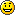### 最新评论（24）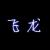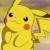ruby的作者在书中就说说他大量参考了lisp等语言的特性，而且，java的自动回收垃圾功能早在lisp那里就有了，lisp可以说是很多语言的始祖，而且在某些领域还是不可替代的语言。

List(1,2,3,4,5,6,7,8,9,0)输出成"88,99,00"试试，在scala里面，
myList.take(7).map(_.toString * 2).mkString(",")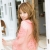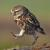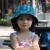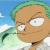C++ 本身 template 也带有函数式的影子, 写起来也很有趣, 在旧标准中使用类重载 () 符号，模仿 lambda 也是比较 trick 的方式, 如果觉得自己的代码中使用该方式比使用传统的方式开发在代码上语义更清晰, 也是值得尝试的.Java 新版 以及 C++11 支持 lambda 也是在改善语言的开发效率, 既然已经支持了, 作者当然会根据自己工作经验推荐大家在合适的地方使用.
Python web 开发里封装的框架也大量使用了装饰器, 那本身就是一种函数式编程方式, 不说Java程序员, 如果有Python 开发经验的,只知道在框架里堆代码，对内部实现机制不了解, 可以先看看. 比如 Python Tornado 框架源码